CAT  >  Test: CAT Quant 2017 (Shift - 1)

# Test: CAT Quant 2017 (Shift - 1)

Test Description

## 34 Questions MCQ Test Quantitative Aptitude (Quant) | Test: CAT Quant 2017 (Shift - 1)

Test: CAT Quant 2017 (Shift - 1) for CAT 2022 is part of Quantitative Aptitude (Quant) preparation. The Test: CAT Quant 2017 (Shift - 1) questions and answers have been prepared according to the CAT exam syllabus.The Test: CAT Quant 2017 (Shift - 1) MCQs are made for CAT 2022 Exam. Find important definitions, questions, notes, meanings, examples, exercises, MCQs and online tests for Test: CAT Quant 2017 (Shift - 1) below.
Solutions of Test: CAT Quant 2017 (Shift - 1) questions in English are available as part of our Quantitative Aptitude (Quant) for CAT & Test: CAT Quant 2017 (Shift - 1) solutions in Hindi for Quantitative Aptitude (Quant) course. Download more important topics, notes, lectures and mock test series for CAT Exam by signing up for free. Attempt Test: CAT Quant 2017 (Shift - 1) | 34 questions in 60 minutes | Mock test for CAT preparation | Free important questions MCQ to study Quantitative Aptitude (Quant) for CAT Exam | Download free PDF with solutions
 1 Crore+ students have signed up on EduRev. Have you?
Test: CAT Quant 2017 (Shift - 1) - Question 1

### Arun's present age in years is 40% of Barun's. In another few years, Arun's age will be half of Barun's. By what percentage will Barun's age increase during this period?

Detailed Solution for Test: CAT Quant 2017 (Shift - 1) - Question 1

Let Arun's current age be A. Hence, Barun's current age is 2.5A

Let Arun's age be half of Barun's age after X years.

Therefore, 2*(X+A) = 2.5A + X

or, X = 0.5A

Hence, Barun's age increased by 0.5A/2.5A = 20%

Test: CAT Quant 2017 (Shift - 1) - Question 2

### A person can complete a job in 120 days. He works alone on Day 1. On Day 2, he is joined by another person who also can complete the job in exactly 120 days. On Day 3, they are joined by another person of equal efficiency. Like this, everyday a new person with the same efficiency joins the work. How many days are required to complete the job?

Detailed Solution for Test: CAT Quant 2017 (Shift - 1) - Question 2

Let the rate of work of a person be x units/day. Hence, the total work = 120x.

It is given that one first day, one person works, on the second day two people work and so on.

Hence, the work done on day 1, day 2... will be x, 2x, 3x, ... respectively.

The sum should be equal to 120x.

120x = x * n(n+1)/2

n2 + n – 240 = 0

n = 15 is the only positive solution.

Hence, it takes 15 days to complete the work.

Test: CAT Quant 2017 (Shift - 1) - Question 3

### An elevator has a weight limit of 630 kg. It is carrying a group of people of whom the heaviest weighs 57 kg and the lightest weighs 53 kg. What is the maximum possible number of people in the group?

Detailed Solution for Test: CAT Quant 2017 (Shift - 1) - Question 3

It is given that the maximum weight limit is 630. The lightest person's weight is 53 Kg and the heaviest person's weight is 57 Kg.

In order to have maximum people in the lift, all the remaining people should be of the lightest weight possible, which is 53 Kg.

Let there be n people.

53 + n (53) + 57 = 630

n is approximately equal to 9.8. Hence, 9 people are possible.

Therefore, a total of 9 + 2 = 11 people can use the elevator.

Test: CAT Quant 2017 (Shift - 1) - Question 4

A man leaves his home and walks at a speed of 12 km per hour, reaching the railway station 10 minutes after the train had departed. If instead he had walked at a speed of 15 km per hour, he would have reached the station 10 minutes before the train's departure. The distance (in km) from his home to the railway station is

Detailed Solution for Test: CAT Quant 2017 (Shift - 1) - Question 4

We see that the man saves 20 minutes by changing his speed from 12 Km/hr to 15 Km/hr. Let d be the distance

Hence,

d/12 – d/15 = 1/3

d/60 = 1/3

d = 20 Km.

Test: CAT Quant 2017 (Shift - 1) - Question 5

Ravi invests 50% of his monthly savings in fixed deposits. Thirty percent of the rest of his savings is invested in stocks and the rest goes into Ravi's savings bank account. If the total amount deposited by him in the bank (for savings account and fixed deposits) is Rs 59500, then Ravi's total monthly savings (in Rs) is

Detailed Solution for Test: CAT Quant 2017 (Shift - 1) - Question 5

Let his total savings be 100x.

He invests 50x in fixed deposits. 30% of 50x, which is 15x is invested in stocks and 35x goes to savings bank.

It is given 85x = 59500

x = 700

Hence, 100x = 70000

Test: CAT Quant 2017 (Shift - 1) - Question 6

If a seller gives a discount of 15% on retail price, she still makes a profit of 2%. Which of the following ensures that she makes a profit of 20%?

Detailed Solution for Test: CAT Quant 2017 (Shift - 1) - Question 6 Let the retail price be M and cost price be C.

Given,

0.85 M = 1.02 C

M = 1.2 C

If he wants 20% profit he has to sell at 1.2C, which is nothing but the retail price.

Test: CAT Quant 2017 (Shift - 1) - Question 7

A man travels by a motor boat down a river to his office and back. With the speed of the river unchanged, if he doubles the speed of his motor boat, then his total travel time gets reduced by 75%. The ratio of the original speed of the motor boat to the speed of the river is

Detailed Solution for Test: CAT Quant 2017 (Shift - 1) - Question 7

Total travel time gets reduced by 75%. So, total travel time becomes 1/4th of the original.
So, s’(New speed) = 4s(Original speed)
Speed of boat : Speed of river = x:1
When the speed of boat is normal
Speed Upstream = x-1
Speed Downstream = x+1
Here, the distance is constant. So, average speed can be found out by the harmonic mean of two speeds = 2ab/ (a+b)
Here, average speed will be 2(x^2-1)/2x = (x^2-1)/x
When the speed of boat is doubled
Speed upstream = 2x-1
Speed downstream = 2x+1
Here, the distance is constant. So, average speed can be found out by the harmonic mean of two speeds = 2ab/(a+b)
Here, average speed will be 2(4x^2-1)/4x = (4x^2-1)/2x
Now, we know that
New speed = 4*Original speed
So,
(4x^2-1)/2x = 4(x^2-1)/x
4x^2 – 1 = 8x^2 – 8
4x^2 = 7
x = √7/2
Ratio = x:1 = √7/2

Test: CAT Quant 2017 (Shift - 1) - Question 8

Suppose, C1, C2, C3, C4, and C5 are five companies. The profits made by C1, C2, and C3 are in the ratio 9: 10: 8 while the profits made by C2, C4, and C5 are in the ratio 18 : 19 : 20. If C5 has made a profit of Rs 19 crore more than C1, then the total profit (in Rs) made by all five companies is

Detailed Solution for Test: CAT Quant 2017 (Shift - 1) - Question 8 Given,

C1 : C2 : C3 = 9 : 10 : 8 ... i

C2 : C4 : C5 = 18 : 19 : 20 ... ii

Let's multiply i by 9 and ii by 5

C1 : C2 : C3 = 81 : 90 : 72

C2 : C4 : C5 = 90 : 95 : 100

Therefore, C1 : C2 : C3 : C4 : C5 = 81 : 90 : 72 : 95 : 100

Given,

100x - 81x = 19

x = 1

Hence, total profit = 100 + 95 + 72 + 90 + 81 = 438

Test: CAT Quant 2017 (Shift - 1) - Question 9

The number of girls appearing for an admission test is twice the number of boys. If 30% of the girls and 45% of the boys get admission, the percentage of candidates who do not get admission is

Detailed Solution for Test: CAT Quant 2017 (Shift - 1) - Question 9 Let the number of girls be 2x and number of boys be x.

Girls getting admission = 0.6x

Boys getting admission = 0.45x

Number of students not getting admission = 3x - 0.6x -0.45x = 1.95x

Percentage = (1.95x/3x) * 100 = 65%

Test: CAT Quant 2017 (Shift - 1) - Question 10

A stall sells popcorn and chips in packets of three sizes: large, super, and jumbo. The numbers of large, super, and jumbo packets in its stock are in the ratio 7: 17: 16 for popcorn and 6: 15: 14 for chips. If the total number of popcorn packets in its stock is the same as that of chips packets, then the numbers of jumbo popcorn packets and jumbo chips packets are in the ratio

Detailed Solution for Test: CAT Quant 2017 (Shift - 1) - Question 10 The ratio of L, S, J for popcorn = 7:17: 16

Let them be 7x, 17x and 16x

The ratio of L, S, J for chips = 6:15: 14

Let them 6y, 15y and 14y

Given, 40x = 35y, x= 7y/8

Jumbo popcor = 16x = 16 * 7y/8= 14y

Hence, the ratio of jumbo popcorn and jumbo chips = 1: 1

Test: CAT Quant 2017 (Shift - 1) - Question 11

In a market, the price of medium quality mangoes is half that of good mangoes. A shopkeeper buys 80 kg good mangoes and 40 kg medium quality mangoes from the market and then sells all these at a common price which is 10% less than the price at which he bought the good ones. His overall profit is

Detailed Solution for Test: CAT Quant 2017 (Shift - 1) - Question 11 Let the cost of good mangoes be 2x per kg. The cost of medium mangoes be x per kg.

CP of good mangoes = 160x

CP of medium mangoes = 40x

His selling price = 0.9*2x = 1.80x

Therefore, total revenue generated by selling all the mangoes = 120*1.8x = 216x

Hence, the profit % =16x/200x * 100= 8%

Test: CAT Quant 2017 (Shift - 1) - Question 12

If Fatima sells 60 identical toys at a 40% discount on the printed price, then she makes 20% profit. Ten of these toys are destroyed in fire. While selling the rest, how much discount should be given on the printed price so that she can make the same amount of profit?

Detailed Solution for Test: CAT Quant 2017 (Shift - 1) - Question 12 Let the cost price be C and the marked price be M.

Given,

0.6 M = 1.2 C

M = 2C

CP of 60 toys = 60C

Now only 50 are remaining.

Hence, M (1 - d) * 50 = 72C

1- d = 0.72

d = .28

Hence 28%

Test: CAT Quant 2017 (Shift - 1) - Question 13

If a and b are integers of opposite signs such that (a+3)2:b = 9:1 and (a-1)2 : (b-1)2 = 4:1, then the ratio a2 + b2 is

Detailed Solution for Test: CAT Quant 2017 (Shift - 1) - Question 13 Since the square root can be positive or negative we will get two cases for each of the equation.

For the first one,

a + 3 = 3b .. i

a + 3 = -3b ... ii

For the second one,

a - 1 = 2(b -1) ... iii

a - 1 = 2 (1 - b) ... iv

we have to solve i and iii, i and iv, ii and iii, ii and iv.

Solving i and iii,

a + 3 = 3b and a = 2b - 1, solving, we get a = 3 and b = 2, which is not what we want.

Solving i and iv

a + 3 = 3b and a = 3 - 2b, solving, we get b = 1.2, which is not possible.

Solving ii and iii

a + 3 = -3b and a = 2b - 1, solving, we get b = 0.4, which is not possible.

Solving ii and iv,

a + 3 = -3b and a = 3 - 2b, solving, we get a = 15 and b = -6 which is what we want.

Thus, a2/b2 = 25/4

Test: CAT Quant 2017 (Shift - 1) - Question 14

A class consists of 20 boys and 30 girls. In the mid-semester examination, the average score of the girls was 5 higher than that of the boys. In the final exam, however, the average score of the girls dropped by 3 while the average score of the entire class increased by 2. The increase in the average score of the boys is

Detailed Solution for Test: CAT Quant 2017 (Shift - 1) - Question 14 Let the average score of girls in the mid semester exam be G.

Therefore the average score of boys in the mid semester exam is G-5.

Hence, the total marks scored by the class is 30*G+20*(G-5) = 50*G – 100

Therefore, the average of the entire class is (50G-100)/50 = G-2

Therefore, the average of girls in the final exam is G-3 and the average of the entire class in the final exam is G.

Hence, the total marks scored by the class is 50G, while the total marks scored by the girls is 30*(G-3)=30G-90

Therefore, the total marks scored by the boys is 50G-(30G-90) = 20G+90

Hence, the average of the boys in the final exam is (20G+90)/20 = G+4.5

Hence, the increase in the average marks of the boys is (G+4.5)-(G-5) = 9.5

Test: CAT Quant 2017 (Shift - 1) - Question 15

The area of the closed region bounded by the equation I x I + I y I = 2 in the two-dimensional plane is

Detailed Solution for Test: CAT Quant 2017 (Shift - 1) - Question 15 The following equation will form a square of side (2√2 )2

The area of the square = (2√2 )2 = 8 units.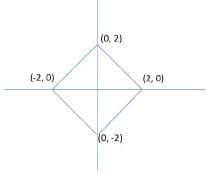Test: CAT Quant 2017 (Shift - 1) - Question 16

From a triangle ABC with sides of lengths 40 ft, 25 ft and 35 ft, a triangular portion GBC is cut off where G is the centroid of ABC. The area, in sq ft, of the remaining portion of triangle ABC is

Detailed Solution for Test: CAT Quant 2017 (Shift - 1) - Question 16 The lengths are given as 40, 25 and 35.

The perimeter = 100

Semi-perimeter, s = 50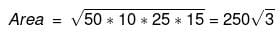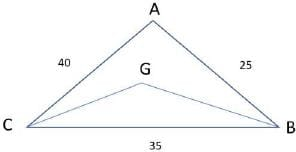The triangle formed by the centroid and two vertices is removed.

Since the cenroid divides the median in the ratio 2 : 1

The remaining area will be two-thirds the area of the original triangle.

Remaining area = 2 / 3 * 250√3 = 500 / √3.

Test: CAT Quant 2017 (Shift - 1) - Question 17

Let ABC be a right-angled isosceles triangle with hypotenuse BC. Let BQC be a semi-circle, away from A, with diameter BC. Let BPC be an arc of a circle centered at A and lying between BC and BQC.If AB has length 6 cm then the area, in sq cm, of the region enclosed by BPC and BQC is

Detailed Solution for Test: CAT Quant 2017 (Shift - 1) - Question 17 The image of the figure is as shown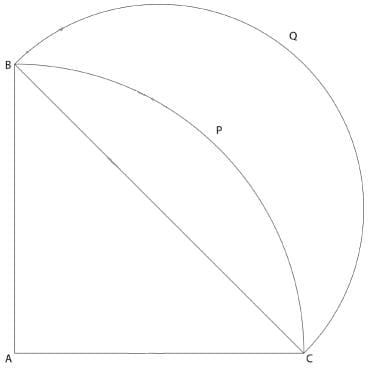AB = AC = 6cm. Thus, BC =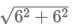= 6√2 cm

The required area = Area of semi-circle BQC - Area of quadrant BPC + Area of triangle ABC

Area of semicircle BQC

Diameter BC = 6√2cm

Radius = 6√2/2 = 3√2 cm

Area = πr2/2 = π*(3√2) /2 = 9π

Area of quadrant BPC

Area = πr2/4 = π * (6)2 /4 = 9π

Area of triangle ABC

Area = 1/2 * 6 * 6 = 18

The required area = Area of semi-circle BQC - Area of quadrant BPC + Area of triangle ABC

= 9π - 9 π + 18 = 18

Test: CAT Quant 2017 (Shift - 1) - Question 18

A solid metallic cube is melted to form five solid cubes whose volumes are in the ratio 1: 1: 8: 27: 27. The percentage by which the sum of the surface areas of these five cubes exceeds the surface area of the original cube is nearest to

Detailed Solution for Test: CAT Quant 2017 (Shift - 1) - Question 18 Let the volumes of the five cubes be x, x, 8x, 27x and 27x.

Let the sides be a, a, 2a, 3a and 3a. (x = a3)

Let the side of the original cube be A.

A3 = x+x+8x+27x+27x

A = 4a

Original surface area = 96a2

New surface area = 6(a2 + a2 + 4a2 + 9a2 + 9a2) = 144a2

Percentage increase = (144 – 96) / 96 * 100 = 50%

Test: CAT Quant 2017 (Shift - 1) - Question 19

A ball of diameter 4 cm is kept on top of a hollow cylinder standing vertically. The height of the cylinder is 3 cm, while its volume is cubic centimeters. Then the vertical distance, in cm, of the topmost point of the ball from the base of the cylinder is

Detailed Solution for Test: CAT Quant 2017 (Shift - 1) - Question 19

The volume of a cylinder is π *r2 *3 = 9π

r = √3 cm.

Radius of the ball is 2 cm. Hence, the ball will lie on top of the cylinder.

Lets draw the figure.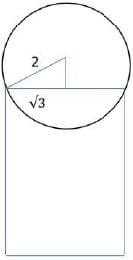Based on the Pythagoras theorem, the other leg will be 1 cm.

Thus, the height will be 3 + 1 + 2 = 6 cm

Test: CAT Quant 2017 (Shift - 1) - Question 20

Let ABC be a right-angled triangle with BC as the hypotenuse. Lengths of AB and AC are 15 km and 20 km, respectively. The minimum possible time, in minutes, required to reach the hypotenuse from A at a speed of 30 km per hour is

Detailed Solution for Test: CAT Quant 2017 (Shift - 1) - Question 20

The length of the altitude from A to the hypotenuse will be the shortest distance.

This is a right triangle with sides 3:4: 5.

Hence, the hypotenuse = = 25 Km.

Length of the altitude = = 12 Km

The time taken = 12/30 * 60 = 24 minutes

Test: CAT Quant 2017 (Shift - 1) - Question 21

Suppose, log3x = log12y = a, where x,y are positive numbers. If G is the geometric mean of x and y, and log6G is equal to

Detailed Solution for Test: CAT Quant 2017 (Shift - 1) - Question 21

We know that log3x = a and log12y = a

Hence, x=3a and y=12aTherefore, the geometric mean of and equals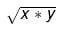This equals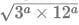= 6a

Hence, G = 6aOr, log6 G = a

Test: CAT Quant 2017 (Shift - 1) - Question 22

If x+1 = x2 and x>0, then 2x4 is

Detailed Solution for Test: CAT Quant 2017 (Shift - 1) - Question 22

We know that x2 – x – 1 =0

Therefore x4 = (x+1)2 = x2 + 2x + 1 = x + 1 + 2x + 1 = 3x + 2

Therefore, 2x4 = 6x + 4

We know that x>0 therefore, we can calculate the value of x to be 1+√5 /2

Hence, 2x4 = 6x + 4 = 3+3√5 +4 = 3√5 + 7

Test: CAT Quant 2017 (Shift - 1) - Question 23

The value of log0.008√5 + log√3 81 – 7 is equal to

Detailed Solution for Test: CAT Quant 2017 (Shift - 1) - Question 23

log0.008√5 + log√3 81 – 7

81 = 34 and 0.008

8/1000 = 23/103 = 1/53 = 5-3

Hence

log0.008√5+ 8 – 7

log5-351/2 + 8 – 7

log50.5/log5-3 + 1

1/-6 + 1

= 5/6

Test: CAT Quant 2017 (Shift - 1) - Question 24

If 92x-1 – 81x-1 = 1944, then x is

Detailed Solution for Test: CAT Quant 2017 (Shift - 1) - Question 24

81x/9 – 81x/81 = 1944

81x * [1/9 – 1/81] = 1944

81x * [8/81] = 243

34x = 39

x = 9/4

Test: CAT Quant 2017 (Shift - 1) - Question 25

The number of solutions (x,y,z) to the equation x – y – z = 25, where x, y, and z are positive integers such that x≤40,y≤12 and z≤12 is

Detailed Solution for Test: CAT Quant 2017 (Shift - 1) - Question 25

x - y - z = 25 and x≤40, y≤12, z≤12

If x = 40 then y + z = 15. Now since both y and z are natural numbers less than 12, so y can range from 3 to 12 giving us a total of 10 solutions. Similarly, if x = 39, then y + z = 14. Now y can range from 2 to 12 giving us a total of 11 solutions.

If x = 38, then y + z = 13. Now y can range from 1 to 12 giving us a total of 12 solutions.

If x = 37 then y + z = 12 which will give 11 solutions.

Similarly on proceeding in the same manner the number of solutions will be 10, 9, 8, 7 and so on till 1.

Hence, required number of solutions will be (1 + 2 + 3 + 4 . . . . + 12) + 10 + 11

= 12*13/2 + 21

78 + 21 = 99

Test: CAT Quant 2017 (Shift - 1) - Question 26

For how many integers n, will the inequality (n-5)(n-10)-3(n-2)≤0 be satisfied?

Detailed Solution for Test: CAT Quant 2017 (Shift - 1) - Question 26

(n-5) (n-10)-3(n-2) ≤ 2

=> n2 − 15n + 50 − 3n + 6 ≤ 0

=> n2 − 18n + 56 ≤ 0

=> (n − 4)(n − 14) ≤ 0

=> Thus, n can take values from 4 to 14. Hence, the required numbers of values are 14 - 4 + 1 = 11.

Positive Mark: 3

Negative Mark: 0

Test: CAT Quant 2017 (Shift - 1) - Question 27

If f1(x) = x2 + 11x + n and f2(x) = x, then the largest positive integer n for which the equation f1(x) = f2(x) has two distinct real roots is

Detailed Solution for Test: CAT Quant 2017 (Shift - 1) - Question 27

f1(x) = x2 + 11x + n and f2(x) = x,

f1(x) = f2(x)

x2 + 11x + n = x

x2 + 10x + n = 0

For this equation to have distinct real roots, b2 - 4ac>0

102> 4n

=> n < 100/4

=> n < 25

Thus, largest integral value that n can take is 24.

Positive Mark: 3

Negative Mark: 0

Test: CAT Quant 2017 (Shift - 1) - Question 28

If a,b,c and d are integers such that a+b+c+d then the minimum possible value of (a-b)2 + (a-c)2+(a-d)2 is

Detailed Solution for Test: CAT Quant 2017 (Shift - 1) - Question 28

For the value of given expression to be minimum, the values of and should be as close as possible. 30/4 = 7.5. Since each one of these are integers so values must be 8, 8, 7, 7. On putting these values in the given expression, we get

(8 − 8)2 + (8 − 7)2 + (8 − 7)2

=> 1 + 1 = 2

Test: CAT Quant 2017 (Shift - 1) - Question 29

Let AB, CD, EF, GH, and JK be five diameters of a circle with center at 0. In how many ways can three points be chosen out of A, B, C, D, E, F, G, H, J, K, and 0 so as to form a triangle?

Detailed Solution for Test: CAT Quant 2017 (Shift - 1) - Question 29

The total number of given points are 11. (10 on circumference and 1 is the center)

So total possible triangles = 11C3 = 165.

However, AOB, COD, EOF, GOH, JOK lie on a straight line. Hence, these 5 triangles are not possible. Thus, the required number of triangles = 165 - 5 = 160

Test: CAT Quant 2017 (Shift - 1) - Question 30

The shortest distance of the point (1/2, 1)from the curve y = I x -1I + I x + 1I is

Detailed Solution for Test: CAT Quant 2017 (Shift - 1) - Question 30 The graph of the given function is as shown below.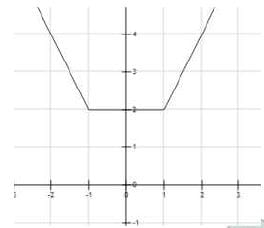We can see that the shortest distance of the point (1/2, 1) will be 1 unit.

Test: CAT Quant 2017 (Shift - 1) - Question 31

If the square of the 7th term of an arithmetic progression with positive common difference equals the product of the 3rd and 17th terms, then the ratio of the first term to the common difference is

Detailed Solution for Test: CAT Quant 2017 (Shift - 1) - Question 31 The seventh term of an AP = a + 6d. Third term will be a + 2d and second term will be a + 16d. We are given that (a + 6d) 2 = (a + 2d) (a + 16d)

=> a2 + 36d2 + 12ad = a2+ 18ad + 32d2

=> 4d2 = 6ad

=>d: a = 3:2

we have been asked about a: d. Hence, it would be 2:3

Test: CAT Quant 2017 (Shift - 1) - Question 32

In how many ways can 7 identical erasers be distributed among 4 kids in such a way that each kid gets at least one eraser but nobody gets more than 3 erasers?

Detailed Solution for Test: CAT Quant 2017 (Shift - 1) - Question 32 We have been given that a + b + c + d = 7

Total ways of distributing 7 things among 4 people so that each one gets at least one = n-1Cr-1 = 6C3 = 20 Now we need to subtract the cases where any one person got more than 3 erasers. Any person cannot get more than 4 erasers since each child has to get at least 1. Any of the 4 childs can get 4 erasers. Thus, there are 4 cases. On subtracting these cases from the total cases we get the required answer. Hence, the required value is 20 - 4 = 16

Test: CAT Quant 2017 (Shift - 1) - Question 33

f(x) = (5x + 2)/ (3x-5) and g(x) = x2 − 2x – 1, then the value of g(f(f(3))) is

Detailed Solution for Test: CAT Quant 2017 (Shift - 1) - Question 33

f (3) = (15 + 2) / (9 - 5) = 17/4

f (f (3)) = (5 * 17 / 4 + 2) / (3 * 17/ 4 – 5) = (93/4)/ (31/4) = 93/31 = 3

g(f (f (3))) = 32 − 3 ∗ 2 − 1 = 2

Test: CAT Quant 2017 (Shift - 1) - Question 34

Let a1, a2,.............,a3n be an arithmetic progression with a1 = 3 and a2 = 7. If a1 + a2 +...+a3n = 1830, then what is the smallest positive integer m such that m (a1 + a2 +...+an) > 1830?

Detailed Solution for Test: CAT Quant 2017 (Shift - 1) - Question 34 a1= 3 and a2= 7. Hence, the common difference of the AP is 4.

We have been given that the sum up to 3n terms of this AP is 1830.

Hence, 1830 = m/2 [2*3 + (m – 1) *4]

=> 1830*2 = m(6 + 4m - 4)

=> 3660 = 2m + 4m2

=> 2m2 + m -1830 = 0

=> (m - 30)(2m + 61) = 0

=> m = 30 or m = -61/2

Since m is the number of terms so m cannot be negative. Hence, must be 30

So, 3n = 30

n = 10

Sum of the first '10' terms of the given AP = 5*(6 + 9*4) = 42*5 = 210

m(a1 +a2 +...+an) > 1830

=> 210m > 1830

=> m > 8.71

Hence, smallest integral value of 'm' is 9.

## Quantitative Aptitude (Quant)

163 videos|152 docs|131 tests
 Use Code STAYHOME200 and get INR 200 additional OFF Use Coupon Code
Information about Test: CAT Quant 2017 (Shift - 1) Page
In this test you can find the Exam questions for Test: CAT Quant 2017 (Shift - 1) solved & explained in the simplest way possible. Besides giving Questions and answers for Test: CAT Quant 2017 (Shift - 1), EduRev gives you an ample number of Online tests for practice

## Quantitative Aptitude (Quant)

163 videos|152 docs|131 tests

### How to Prepare for CAT

Read our guide to prepare for CAT which is created by Toppers & the best Teachers